$$\renewcommand{\tr}{{#1}^{\mkern-1.5mu\mathsf{T}}}$$ $$\renewcommand{\ve}{\mathbf{#1}}$$ $$\renewcommand{\sv}{\boldsymbol{#1}}$$ $$\renewcommand{\pop}{\mathcal{#1}}$$ $$\renewcommand{\samp}{\mathcal{#1}}$$ $$\renewcommand{\imply}{\Longrightarrow}$$ $$\renewcommand{\given}{~\vert~}$$ $$\renewcommand{\suchthat}{~:~}$$ $$\renewcommand{\widebar}{\overline{#1}}$$ $$\renewcommand{\wig}{\tilde{#1}}$$ $$\renewcommand{\bigwig}{\widetilde{#1}}$$ $$\renewcommand{\field}{\mathbb{#1}}$$ $$\renewcommand{\Reals}{\field{R}}$$ $$\renewcommand{\abs}{\left\lvert ~{#1} ~\right\rvert}$$ $$\renewcommand{\size}{\left\lvert {#1} \right\rvert}$$ $$\renewcommand{\tr}{{#1}^{\mkern-1.5mu\mathsf{T}}}$$ $$\renewcommand{\norm}{\left|\left|{#1}\right|\right|}$$ $$\renewcommand{\intersect}{\cap}$$ $$\renewcommand{\union}{\cup}$$

The other vignettes emphasize the use of eikosograms in understanding probability and relations between categorical variates. Here, we just give a few example of the use of eikosograms in data analysis.

Because categorical data can present itself in a variety of forms (e.g. tables, factors in a data frame, etc.), the illustrations centre around different types of data structure

tables

Contingency tables (multi-way tables or “data cubes”) are possibly the most common arrangement of categorical data. They are used, for example, in the introductory vignette.

Here, we present a simple analysis of the University of California (Berkeley) graduate school admissions.

Note that eikosograms are grid objects (eikos() returns a grob) and so may be manipulated as any other. For example, several eikosograms could appear in the same display using grid.arrange() (from the gridExtra package).

library(eikosograms)
library(gridExtra)

University administrators were apparently alarmed (as they often are) when they looked at the numbers and saw the relationship between gender and admission rates.

yaxs = FALSE, xaxs = FALSE,
draw = FALSE)
yaxs = FALSE, xaxs = FALSE,
draw = FALSE)
# Using the gridExtra package, draw these in a single plot
grid.arrange(e1, e2, nrow = 1)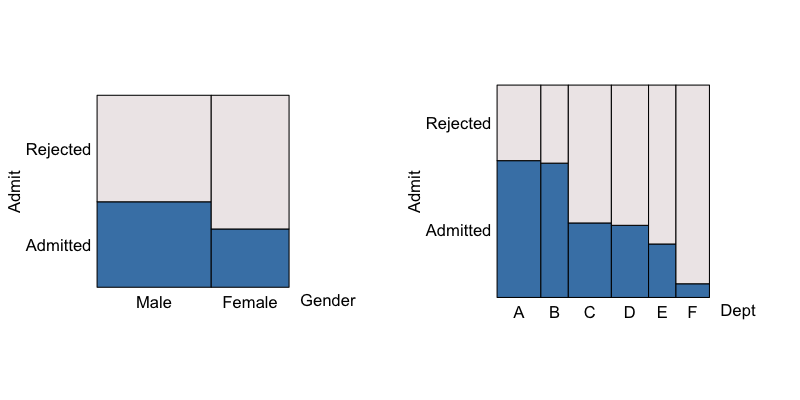At left we see that male applicants are admitted more frequently than female applicants, suggesting a possible gender bias to university administrators. At right, we see that some departments admit more applicants than others, suggesting some may be more difficult to get into than others.

When all three variates are considered together, the true story emerges

yaxs = FALSE, xaxs = FALSE,
lock_aspect = FALSE,
xlab_rot = 90, xvals_size = 8,
ispace = list(bottom = 15))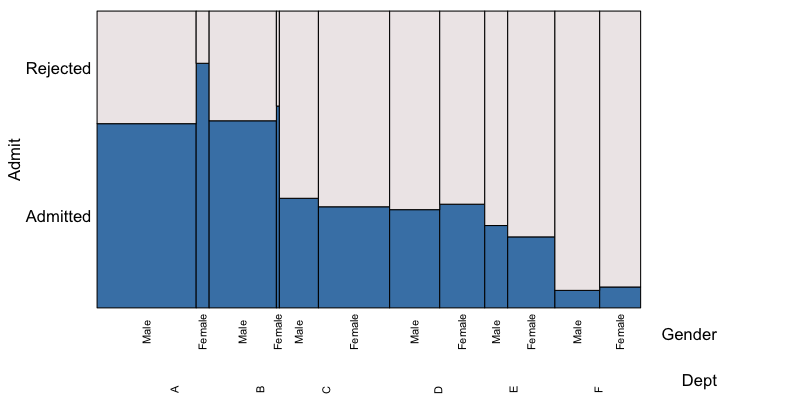In all departments but C and E (which are near equal), a greater proportion of female applicants were accepted than males. This is a famous example of Simpson’s paradox. As can be seen perhaps more clearly from the following eikosogram, the reason for the apparent favouritism towards male applicants occured only because a great many more males than females applied to those departments with the highest admission rates. And in those departments (A and B) the admission rates for females was much higher than that for males!

eikos(Gender ~ Dept, data = UCBAdmissions, yprobs = seq(0,1,0.25), xaxs = FALSE)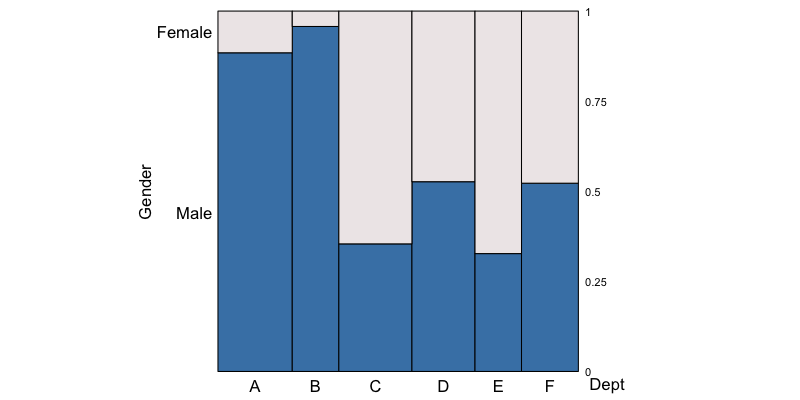Female applicants outnumber male applicants only in departments C and E.

cross tabulation

Sometimes the counts appear as a column in a spreadsheet (or data.frame). Here we consider the counts for habitat type of adult male anolis lizards from Bimini. These are taken frpm Schoener (1968) and are saved here as a text file.

lizards
##       species perch_height_ft perch_diameter_inches count
## 1      sagrei            >= 3               <= 4.25    15
## 2      sagrei            >= 3                > 4.25    18
## 3      sagrei             < 3               <= 4.25    48
## 4      sagrei             < 3                > 4.25    84
## 5 augusticeps            >= 3               <= 4.25    21
## 6 augusticeps            >= 3                > 4.25     1
## 7 augusticeps             < 3               <= 4.25     3
## 8 augusticeps             < 3                > 4.25     2

As can be seen, there are three categorical variates, the species of the anolis lizard, the height (in feet) of where it was observed, and the diameter (in inches) of the branch on which it was perched. The number of such observations is given as the fourth variable, count.

For example, this can be a very convenient form of the data for selecting subsets, particularly for large data sets. Here we might separate the two species:

sagrei <- lizards[lizards$species == "sagrei", -1] augusticeps <- lizards[lizards$species == "augusticeps", -1]

Following Fienberg (1980), we might first consider the relation between perch height and perch diameter for the sagrei species. To look at the eikosigram, these need to be turned into a table using the cross-tabulation xtabs() function:

sagreiTable <- xtabs(count ~ perch_height_ft + perch_diameter_inches, data = sagrei)

and draw it

eikos(perch_height_ft ~ perch_diameter_inches, data = sagreiTable,
main = "Habitat of adult male anolis sagrei lizards")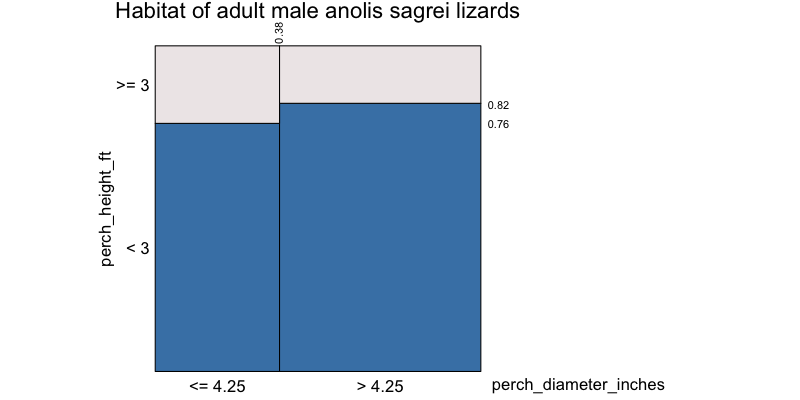The two heights of the bars are very nearly the same, suggesting that the height and the diameter of the perch might be independent variates for the anolis sagrei lizard of Bimini. Indeed, a formal test of independence gives no evidence against this hypothesis.

chisq.test(sagreiTable)
##
##  Pearson's Chi-squared test with Yates' continuity correction
##
## data:  sagreiTable
## X-squared = 0.57934, df = 1, p-value = 0.4466

Now, were we to consider the same for anolis augusticeps, first the table

augusticepsTable <- xtabs(count ~ perch_height_ft + perch_diameter_inches, data = augusticeps)

and then its probability picture

eikos(perch_height_ft ~ perch_diameter_inches, data = augusticepsTable,
main = "Habitat of adult male anolis augusticeps lizards")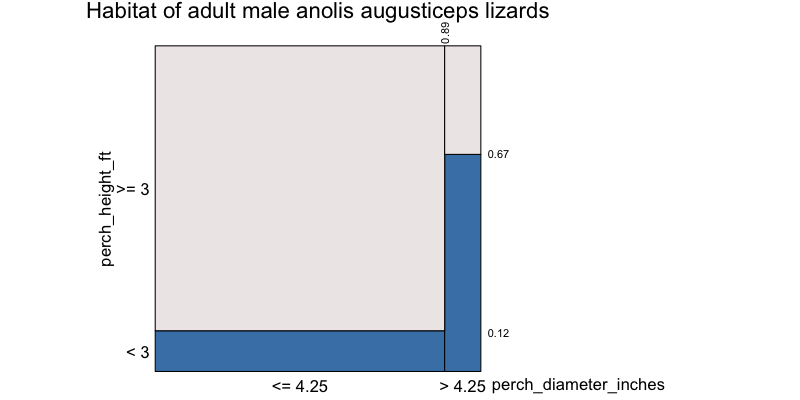Clearly, the bars do not appear to be of equal height. That the eikosogram is not even nearly flat is suggestive that perch height and diameter are not independent for the species anolis augusticeps. However, there needs to be some caution exercised here. As with anolis sagrei, one needs to formally test the hypothesis of independence for a sample of counts. There are so few observations (1,2, and 3 in three cells and 21 in the largest) that any formal test will also show no evidence against the hypothesis of independence in this case. (This includes a line up test using eikosograms as the display for each generated data set!)

All three variates could be examined at once by producing the three way cross-classified table and the corresponding eikosograms. Following Fienberg (1980), we first consider the relation between perch height and perch diameter for the sagrei species of the anolis lizard. The table

lizardsTable <- xtabs(count ~ species + perch_height_ft + perch_diameter_inches,
data = lizards)

and its picture

eikos(species ~ . , data = lizardsTable, lock_aspect = FALSE, yprobs = seq(0,1, 0.1))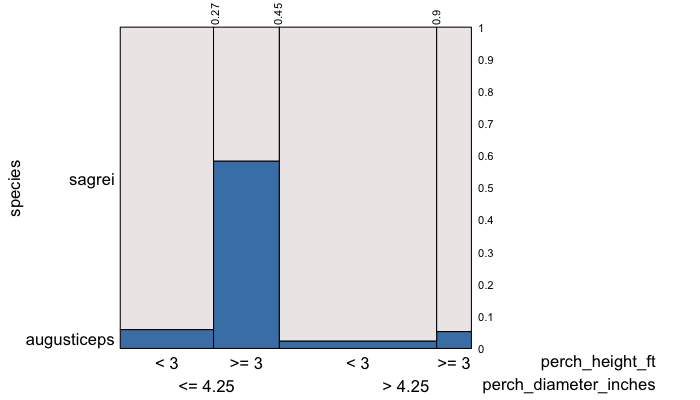listings (data frame rows)

The final way in which categorical data is commonly presented is in a listing (e.g. data.frame) where each row corresponds to a single occurrence of that combination of values for the variates.

For example, consider the mtcars dataset in R, the first few rows of which are

mpg cyl disp hp drat wt qsec vs am gear carb
Mazda RX4 21.0 6 160 110 3.90 2.620 16.46 0 1 4 4
Mazda RX4 Wag 21.0 6 160 110 3.90 2.875 17.02 0 1 4 4
Datsun 710 22.8 4 108 93 3.85 2.320 18.61 1 1 4 1
Hornet 4 Drive 21.4 6 258 110 3.08 3.215 19.44 1 0 3 1
Hornet Sportabout 18.7 8 360 175 3.15 3.440 17.02 0 0 3 2
Valiant 18.1 6 225 105 2.76 3.460 20.22 1 0 3 1

Although all of these variables are numeric, it is clear that many of them could be treated as categorical. For example, vs is a simple indicator variable indicating the shape of the engine block – 0 if it the cylinders are arranged in a “V” pattern and 1 if they are aligned in a straight line. This variable might instead have been represented as a factor. The same could be said of the variable am which is also a binary variable indicating 0 for an automatic transmission and 1 for a manual.

To make the point, we replace these two numeric variables by factors.

mtcars$vs <- factor(mtcars$vs, labels = c("V-shaped", "straight"))
mtcars$am <- factor(mtcars$am, labels = c("automatic", "manual"))

so that the first few rows of mtcars now look like

mpg cyl disp hp drat wt qsec vs am gear carb
Mazda RX4 21.0 6 160 110 3.90 2.620 16.46 V-shaped manual 4 4
Mazda RX4 Wag 21.0 6 160 110 3.90 2.875 17.02 V-shaped manual 4 4
Datsun 710 22.8 4 108 93 3.85 2.320 18.61 straight manual 4 1
Hornet 4 Drive 21.4 6 258 110 3.08 3.215 19.44 straight automatic 3 1
Hornet Sportabout 18.7 8 360 175 3.15 3.440 17.02 V-shaped automatic 3 2
Valiant 18.1 6 225 105 2.76 3.460 20.22 straight automatic 3 1

The eikosogram of these two factors can be compared without contructing a table; eikos() does the counting of matching rows to produce the picture.

eikos(am ~ vs, data = mtcars)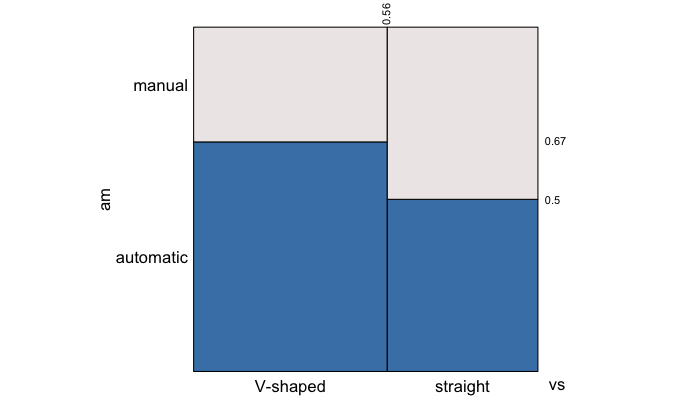This matching does not depend on the variables being factors. Any variable in a data.frame would do and would be treated as if it were categorical. In the mtcars data, there are several variables that are effectively ordinal categorical variates, namely cyl, gear, and carb.

These too could be explored using eikosograms. Transmission type versus number of cylinders

eikos(am ~ cyl, data = mtcars)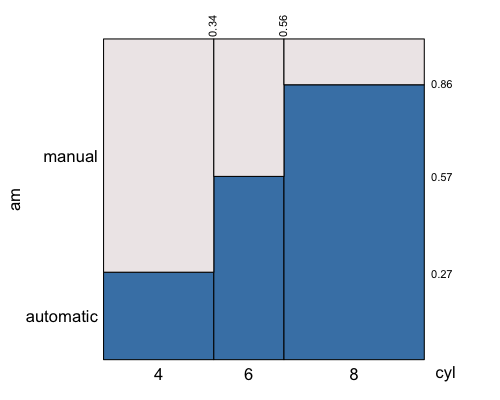and number of forward gears versus number of cylinders

eikos(gear ~ cyl, data = mtcars)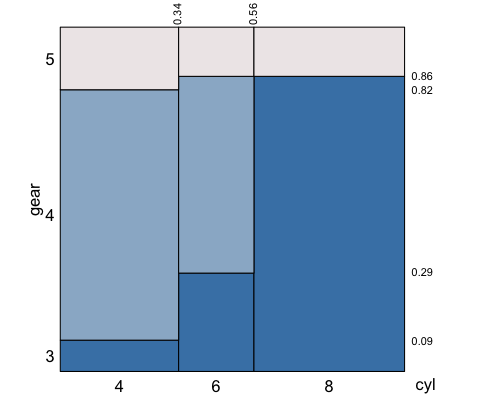fitted models

There are numerous types of models that can be fitted to categorical data. One of the more popular are generalized linear models. For example, we might fit a log-linear model to the lizards habitat data.

fittedModel <- glm(count ~ species + perch_height_ft,
family="poisson",
data = lizards)

This is a “main effects” only model and contains no interaction term. To see what this model asserts about the relationship between species and perch_height, we need to use the fitted.values from the model as the expected counts. A new data.frame is constructed from the fit as follows:

# Can simply append the fitted values to the lizards to get a new data frame
lizardsFit <- data.frame(lizards,  fit = fittedModel$fitted.values) # and create the table fitTable <- xtabs(fit ~ species + perch_height_ft, data=lizardsFit) The eikosogram corresponding to the model fitted to these two variables is eikos("species", "perch_height_ft", data = fitTable)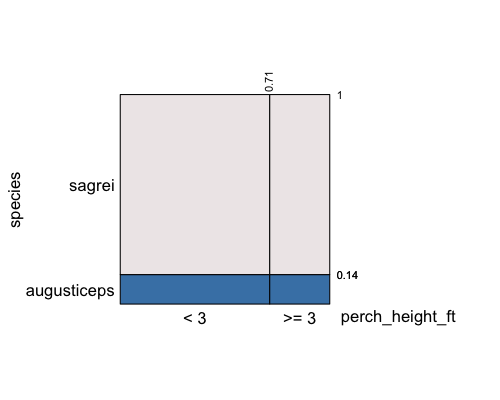from which we can see that the model is asserting that species and perch_height_ft are independently distributed. In log-linear modelling, independence and conditional independence are asserted by the interaction terms which appear in these (hierarchical) models. For example, the following model forces independence between perch_height_ft and perch_diameter_inches, conditional on species. fittedModel3way <- glm(count ~ species + perch_height_ft + perch_diameter_inches + perch_height_ft * species + perch_diameter_inches * species, family="poisson", data = lizards) The absence of the terms perch_height_ft * perch_diameter_inches and perch_height_ft * perch_diameter_inches * species means that when species is held fixed, the terms perch_height_ft and perch_diameter_inches are sepable in the model. Hence they are conditionally independent. As before, we can see this by viewing the eikosogram for the fitted values. # Can simply append the fitted values to the lizards to get a new data frame lizardsFit3way <- data.frame(lizards, fit = fittedModel3way$fitted.values)
# and create the table
fitTable3way <- xtabs(fit ~  species + perch_height_ft + perch_diameter_inches,  data=lizardsFit3way)
# and show the eikosograms
eikos(y = "perch_diameter_inches", x = c("perch_height_ft", "species"), data = fitTable3way,
xlab_rot = 30)